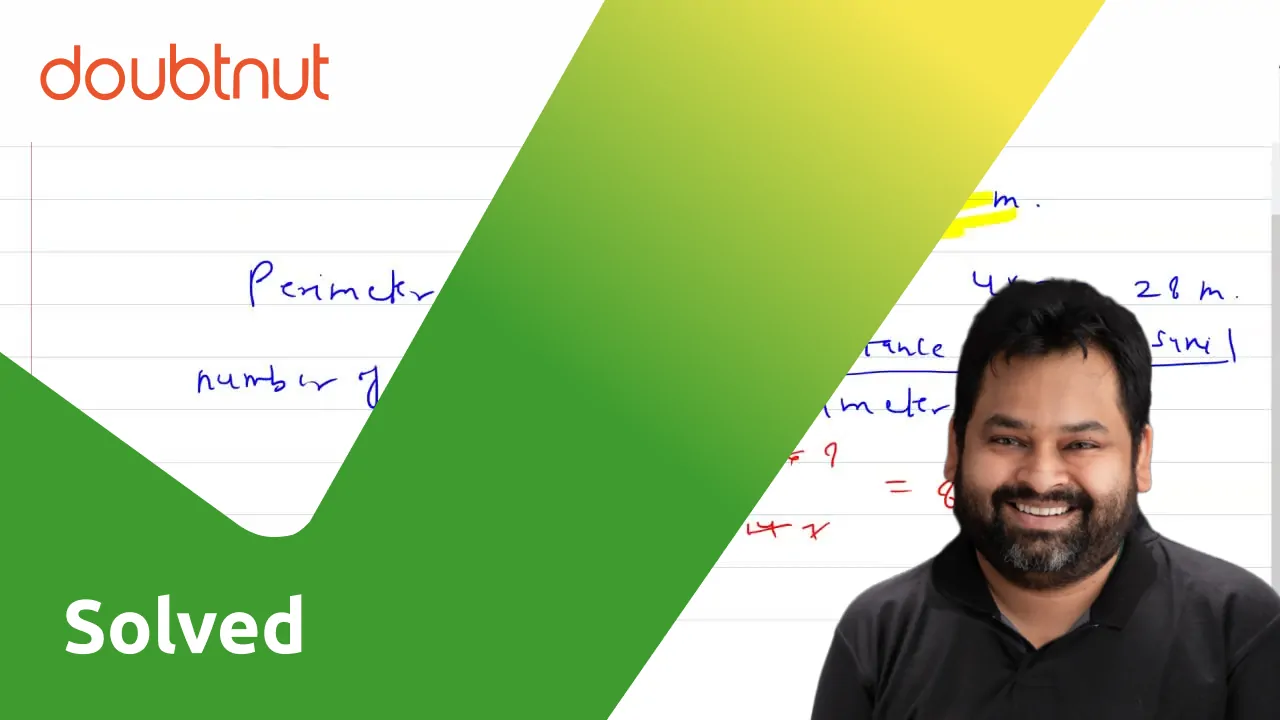Doubtnut App - Get 50 Lakh+ video solutions better than GoogleInstall Now

# The area of a square field is 49m. How many times Sunil has to run around the squrare field to cover 224cm ?

Text Solution
Verified by Experts

## Let side of the square field be a. a2=49 a=√49=7m Distance covered by Sunil in one round =Perimeter of a square =4×7=28cm =Total distanceDistance covered in one round =22428=8